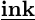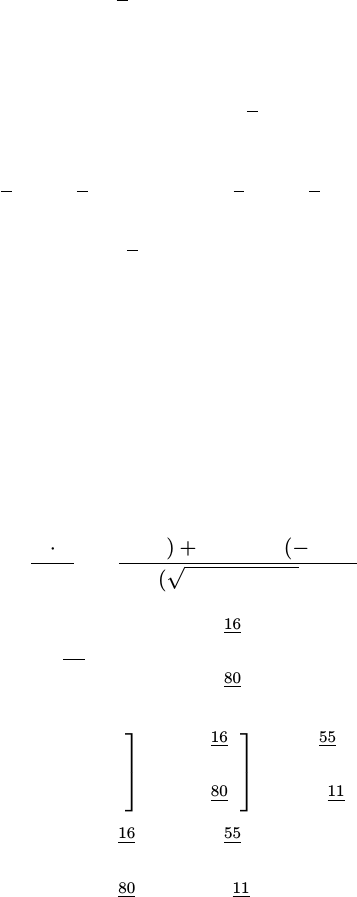Study Guides (390,000)
CA (150,000)
York (10,000)
MATH (500)
Final

# MATH 1025 Study Guide - Final Guide: Elementary Matrix, Parallelogram Law, Gaussian EliminationExam

Department
Mathematics and Statistics
Course Code
MATH 1025
Professor
Asia Weiss
Study Guide
Final

This preview shows pages 1-2. to view the full 7 pages of the document.YORK UNIVERSITY
Faculty of Science
Department of Mathematics and Statistics
MATH 1025 3.00 – Section N
Test #1 February 01, 2019
Carefully read and complete the information below in ink.
SOLUTIONS
INSTRUCTIONS:
1. This is a closed book, closed notes test, duration – 50 minutes.
2. Calculators, Internet connected devices or other aids are NOT permitted.
3. There are 6 questions on pages 2–7. Answer the all questions.
solutions only on pages 2–7. Check the backs of the sheets for questions.
5. Do the easiest questions ﬁrst, GOOD LUCK!
6. Remain seated until we collect all the test papers.
I. Raguimov

Only pages 1-2 are available for preview. Some parts have been intentionally blurred.1. (4 + 5 pts)
(a) Consider the triangle with vertices A, B and C. If Eis the midpoint of the side BC,
show that
AE =1
2(
AB +
AC).
AE =
AC +
CE =
AC +1
2
CB.
So,
AE =1
2
AC +1
2(
AC +
CB) = 1
2
AC +1
2
AB.
AE =1
2(
AB +
AC).
OR EQUIVALENT using the Parallelogram Law.
(b) let
u=
3
1
7
and
v=
1
0
5
.Write ~u =~uk+~u,where ~ukis parallel to ~v and ~u
is orthogonal to ~v.
~uk=
proj ~v ~u =~u ·~v
k~vk2~v =(3)(1) + (1)(0) + (7)(5)
(12+ 02+ 52)2
1
0
5
=16
13
1
0
5
=
16
13
0
80
13
.
Then
~u=~u ~uk=
3
1
7
16
13
0
80
13
=
55
13
1
11
13
.
~u =
16
13
0
80
13
+
55
13
1
11
13
.
Continues...